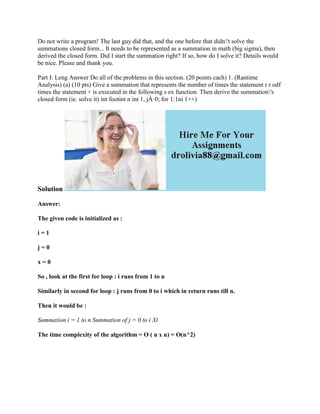Successfully reported this slideshow.

# Do not write a program! The last guy did that- and the one before that.docx×

# Do not write a program! The last guy did that- and the one before that.docx

Do not write a program! The last guy did that, and the one before that didn\'t solve the summations closed form... It needs to be represented as a summation in math (big sigma), then derived the closed form. Did I start the summation right? If so, how do I solve it? Details would be nice. Please and thank you.
Part I: Leng Answer Do all of the problems in this section. (20 points cach) 1. (Rantime Analysis) (a) (10 pts) Give a summation that represents the number of times the statement r r odf times the statement + is executed in the following s ex function. Then derive the summation\'s closed form (ie. solve it) int footint n int 1, jÂ·0; for 1:1ni 1++)
Solution
The given code is initialized as :
i = 1
j = 0
x = 0
So , look at the first for loop : i runs from 1 to n
Similarly in second for loop : j runs from 0 to i which in return runs till n.
Then it would be :
Summation i = 1 to n Summation of j = 0 to i Xi
The time complexity of the algorithm = O ( n x n) = O(n^2)
.

Do not write a program! The last guy did that, and the one before that didn\'t solve the summations closed form... It needs to be represented as a summation in math (big sigma), then derived the closed form. Did I start the summation right? If so, how do I solve it? Details would be nice. Please and thank you.
Part I: Leng Answer Do all of the problems in this section. (20 points cach) 1. (Rantime Analysis) (a) (10 pts) Give a summation that represents the number of times the statement r r odf times the statement + is executed in the following s ex function. Then derive the summation\'s closed form (ie. solve it) int footint n int 1, jÂ·0; for 1:1ni 1++)
Solution
The given code is initialized as :
i = 1
j = 0
x = 0
So , look at the first for loop : i runs from 1 to n
Similarly in second for loop : j runs from 0 to i which in return runs till n.
Then it would be :
Summation i = 1 to n Summation of j = 0 to i Xi
The time complexity of the algorithm = O ( n x n) = O(n^2)
.